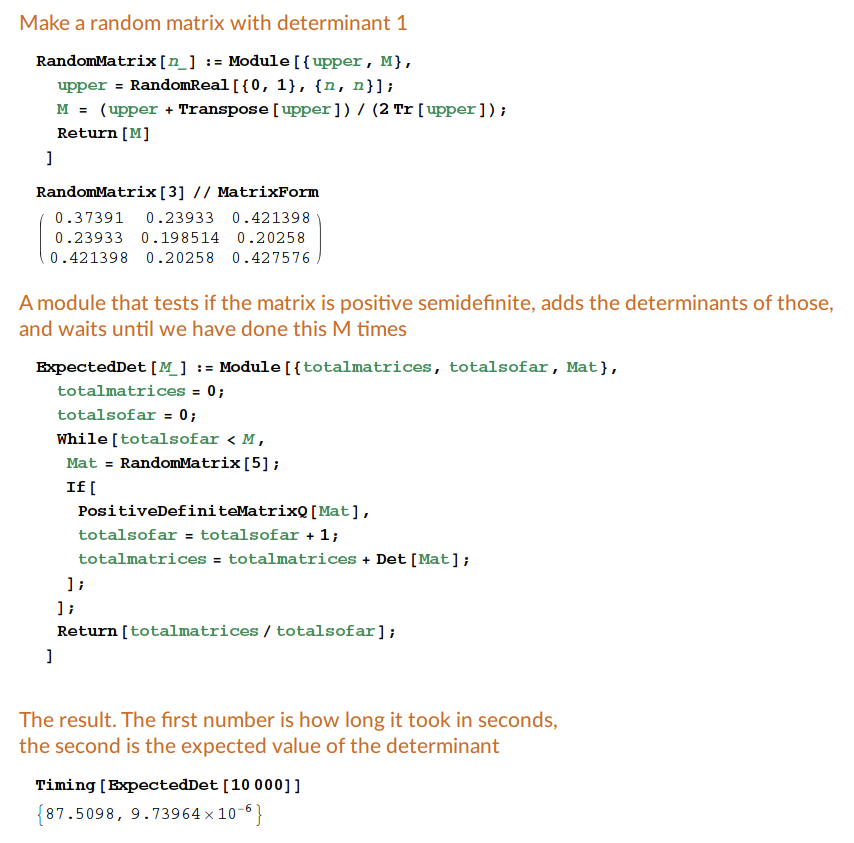# Problem 7

Draw 10000 samples from the set of positive definite symmetric $$5 \times 5$$-matrices with trace one. Use the distribution that Paul Breiding likes best. What is the expected value for the determinant of your matrices?

## Solution

### Mathematica

Make a random matrix with determinant 1:

RandomMatrix[n_] := Module[{upper, M},
upper = RandomReal[{0, 1}, {n, n}];
M = (upper + Transpose[upper])/(2 Tr[upper]);
Return[M]
]

RandomMatrix // MatrixForm


A module that tests if the matrix is positive semidefinite, add the determinants of those, and waits until we have done this M times:

ExpectedDet[M_] := Module[{totalmatrices, totalsofar, Mat},
totalmatrices = 0;
totalsofar = 0;
While[totalsofar < M,
Mat = RandomMatrix;
If[
PositiveDefiniteMatrixQ[Mat],
totalsofar = totalsofar + 1;
totalmatrices = totalmatrices + Det[Mat];
];
];
Return[totalmatrices/totalsofar];
]


The result. The first number is how long it took in seconds, the second is the expected value of the determinant:

Timing[ExpectedDet]